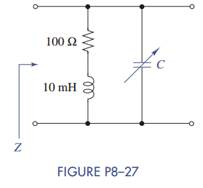Create an Account

Home / Questions / A capacitor C is connected in parallel with a resistor R. Select values of R and C so that the...

A capacitor C is connected in parallel with a resistor R. Select values of R and C so that the...

1. A capacitor C is connected in parallel with a resistor R. Select values of R and C so that the equivalent impedance of the parallel combination is 300 − j400 Ω at ω = 1 Mrad/s.

2. The circuit in Figure P8–27 is excited by a 1 krad/s sinusoidal source. As the circuit’s designer, select a capacitor C such that the impedance Z looking into the circuit is all real.Jul 27 2020 View more View LessSubscribe To Get Solution